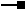# IDeviationInterval Interface

Provides access to members that control the classification methods that require a standard deviation based range.

## Description

IDeviationIntervalis implemented by StandardDeviation, and is used to set the standard deviation interval. Set values on this interface after calling the SetHistogram method on the IClassify interface. Then, you can call IClassify.Classify.

## Members

Name DescriptionDeviationInterval The deviation interval (1/4 <= value <= 2).Mean The mean value.StandardDev The standard deviation.

### IDeviationInterval.DeviationInterval Property

The deviation interval (1/4 <= value <= 2).

``````Public Property DeviationInterval As Double
``````
``````public double DeviationInterval {get; set;}
``````

#### Description

DeviationInterval is the interval for the standard deviation classification. The interval is expressed as a percentage of 1 standard deviation. The value range that may be entered is 0.25 to 1.0.

### IDeviationInterval.Mean Property

The mean value.

``````Public WriteOnly Property Mean
``````
``````public void Mean {set;}
``````

#### Description

Mean sets the mean value for the Standard Deviation classification.

### IDeviationInterval.StandardDev Property

The standard deviation.

``````Public WriteOnly Property StandardDev
``````
``````public void StandardDev {set;}
``````

#### Description

StandardDev sets the Standard Deviation value for the Standard Deviation classification

## Classes that implement IDeviationInterval

Classes Description
StandardDeviation Defines a standard deviation classification method.